# Turning effect of a force

### Guest Account

Attempt Form Two Physics Questions
Guest Account

# Turning Effect of a Force

## Turning Effects

- The turning effect of a body is called the moment of that force.
- The turning effect produced depends on both the size of the force and the distance from the pivot.
- The moment of a force about a point is the product of the force applied and the perpendicular distance from the pivot (or turning point) to the line of action of the force.
Hence, Moments of a force = Force x perpendicular distance from pivot.

### The Law of Moments

- It states that when a body is in balance or in equilibrium, the sum of the clockwise moments equals the sum of anti-clockwise moments.
- The SI units of the moments of a force is Newton metre (Nm).

Example 1
1. A uniform rod of negligible mass balances when a weight of 3 N is at A, weight of 3 N is at B and a weight of W is at C. What is the value of weight W?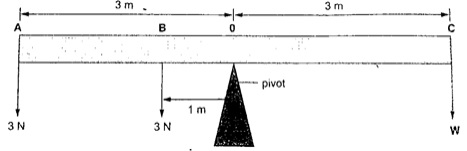Solution
Taking moments about the fulcrum, O then
Anti-clockwise moments = (3 x 1) + (3 x 3)
= 3 + 9 = 12 Nm
Anti-clockwise moments = clockwise moments
3 W = 12 Nm
W = 4 N

Example 2
2. The following bar is of negligible weight. Determine the value of ‘x’ if the bar is balanced.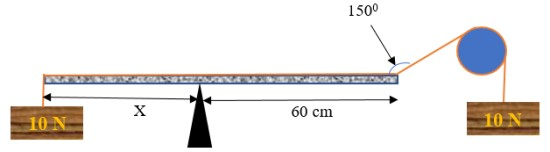Solution
The distance from the turning point to the line of action can be determined as;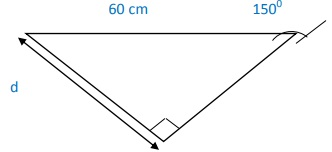Clockwise moments = 10 × 30 = 300 N cm,
Anticlockwise moments = 10 × ‘x’ = 10 x. N cm.
Using the principle of moments
Anti-clockwise moments = clockwise moments
10 x = 300, hence x = 30 cm.

Example 3
3. Study the diagram below and determine the value of X and hence the length of the bar.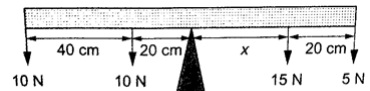Solution
Clockwise moments = 15x N + 5(X x 20) N
Anticlockwise moments = (20 x 10) + (60 × 10) N cm = 800 N cm.
Anti-clockwise moments = clockwise moments
800 N cm = 15X + 5X + 100
800 n cm = 20X + 100
20X = 700
X = 35 cm.
Therefore, the length of the bar = 40 + 20 + 35 + 20 = 115 cm.

## The Lever

-A lever is any device which can turn about a pivot or fulcrum.
-The applied force is called the effort and is used to overcome the resisting force called the load.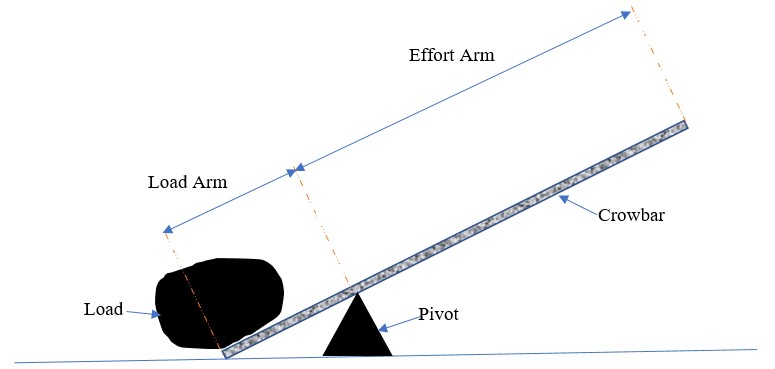Example 4
Consider the following diagram. (The bar is of negligible mass). Determine the effort applied.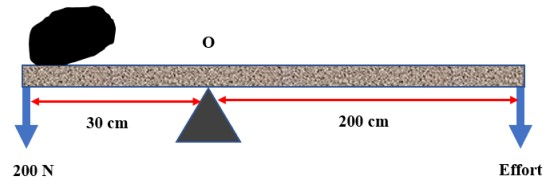Solution
Taking moments about O. Then, clockwise moments = effort x 200 cm.
Anticlockwise moments = 200 x 30 cm.
Effort = (200 x 30)/ 200 = 30 N.